# Using the Model-Based Methods (Advanced Signal Processing Toolkit)

LabVIEW 2014 Advanced Signal Processing Toolkit Help

Edition Date: June 2014

Part Number: 372656C-01

»View Product InfoDownload Help (Windows Only)

Model-based methods assume that a time series is the response from a linear system stimulated by white noise, as shown in the following figure, where white noise et is the stimulus, H(z) is the linear system, and xt is the response time series. Correspondingly, the power spectral density (PSD) of the response time series is the frequency response function (FRF) of the linear system.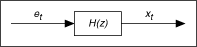In general, you can describe a linear system H(z) with an AR, MA, or ARMA model. To create such a model for a time series, you can estimate the model coefficients first and then use the estimated model coefficients to compute the PSD of the time series. For example, the PSD based on an ARMA model is computed by the following equation: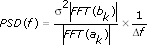where σ2 is the noise variance of the estimated ARMA model of a time series ak is the AR coefficients of the ARMA model that define the poles of the model bk is the MA coefficients of the ARMA model that define the zeros of the model Δf is the frequency interval of the PSD

The AR and MA models are the subsets of the ARMA model, so you also can compute the PSD of a time series based on the AR or MA model from the previous equation by setting the corresponding model coefficients ak or bk to zero.

Use the TSA AR Spectrum VI and the TSA ARMA Spectrum VI to compute the PSD of a time series with model-based methods.

The PSD based on each model has specific characteristics. The following figure shows an AR model-based PSD for a signal from a system with two pairs of poles, which cause the peaks in the PSD at about 0.17 and 0.21 Hz, and one pair of zeros, which cause the valley in the PSD at about 0.25 Hz. In the following figure, the AR model gives a good estimation for peaks and a poor estimation for valleys in the PSD.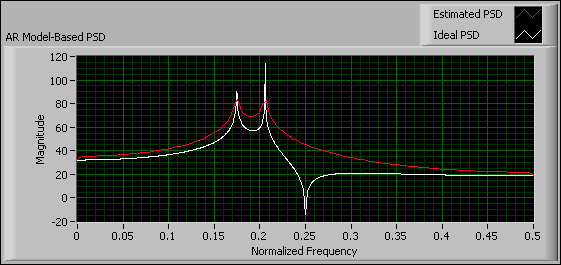The following figure shows an MA model-based PSD, which gives a good estimation for valleys and a poor estimation for peaks.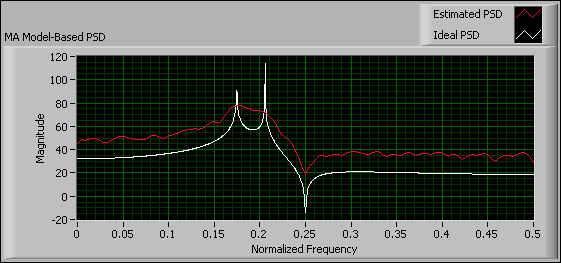The following figure shows an ARMA model-based PSD, which gives the best overall estimation.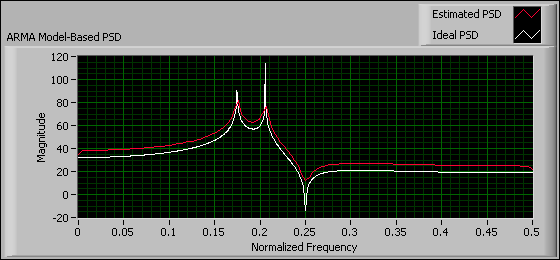In parametric modeling applications, you usually use the AR model-based method instead of the MA or ARMA methods to estimate the PSD of a time series, because this method computes the model coefficients through a linear equation. For real-valued time series, the AR model order is set to at least twice the number of frequency peaks that you want to analyze. The estimated PSD shows more details about the frequency peaks with a large AR model order; however, a much higher AR model order can lead to spurious peaks in the resulting PSD.